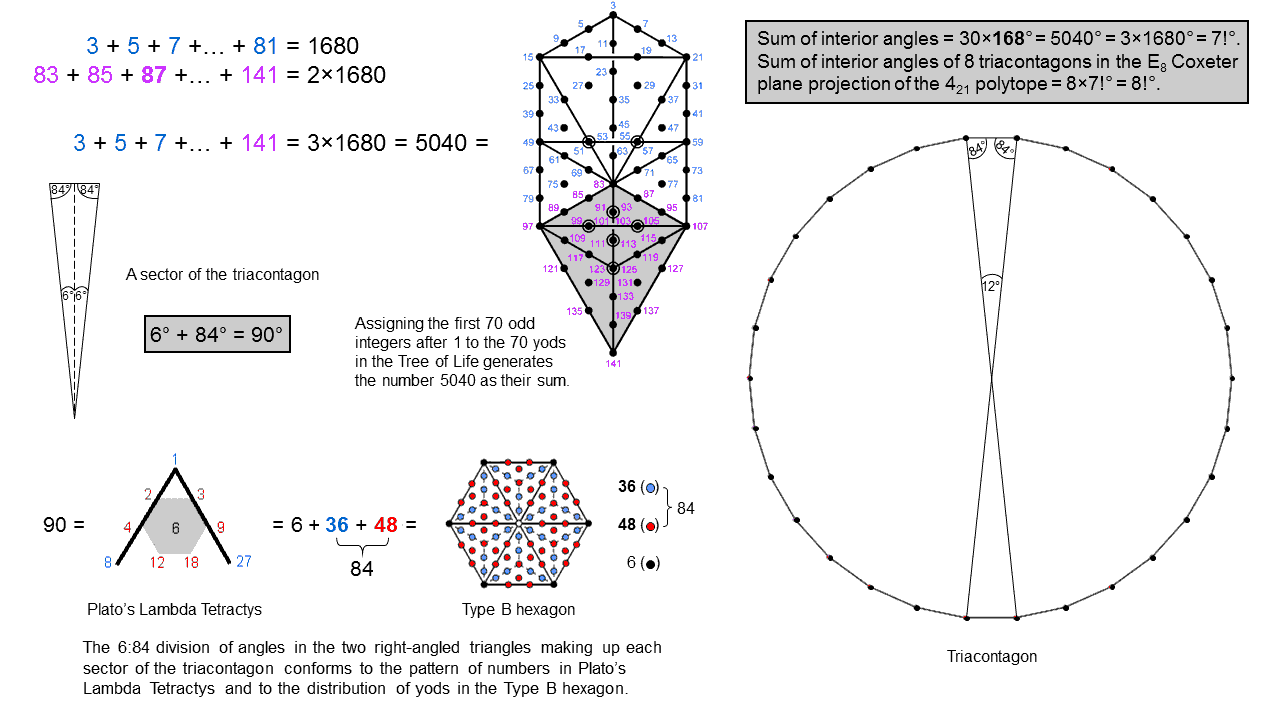Home

 << Previous    1    3  4  5  ...17    Next >>

2. The Lambda Tetractys pattern of the interior & vertex angles of the triacontagonThe angles in each half-sector of the triacontagon conform to the pattern of numbers in the Lambda Tetractys.

The two right-angled triangles making up each sector of the triacontagon contain the angles 90, 84 & 6. The so-called "Lambda Tetractys" is the tetractys array of 10 numbers two sides of which contain the first 4 members of the two geometric series:

1, 2, 4, 8, 16....

1, 3, 9, 27, 81....

They form the sides of what is known to historians of science as "Plato's Lambda" (see Plato's Lambda). Familiar to students of music theory as the numbers whose ratios generate the tone ratios of the Pythagorean musical scale, the 10 numbers add up to 90. The integer at the centre of the Lambda Tetractys is 6. The 9 integers surrounding it add up to 84. The angles in every sector of the 8 triacontagons in the E8 Coxeter plane projection of the 421 polytope are an example of the 6:84 division in the archetypal pattern of the Lambda Tetractys. It is evidence that the symmetry group E8×E8 associated by theoretical physicists with one of the 5 types of superstring is an intrinsic feature of the universe. It is also an illustration of the Hermetic principle "As above, so below" because, in the context of the 91 Trees of Life making up CTOL, there are 90 Trees of Life above the lowest one, of which the lowest 6 Trees map the six subplanes of the physical plane above the lowest one and the remaining 84 Trees map the 84 subplanes above the physical plane (see here).

According to the Tetrad Principle postulated by the author in Article 1, the fourth member of a class of mathematical objects embodies universal parameters of holistic systems embodying the divine archetypes. The hexagon is the fourth type of regular polygon. The Type B hexagon contains 91 yods. It is therefore a polygonal representation of CTOL. Its central yod denotes the lowest Tree in CTOL and the 90 yods surrounding it denote the 90 higher Trees. They comprise:

• 6 black corners of the hexagon;
• 48 red yods either lining sides of its sectors, are corners of tetractyses or their centres;
• 36 blue hexagonal yods that line internal sides of tetractyses.

This division corresponds to the integer 6 at the centre of the Lambda Tetractys, the sum (48) of the 6 integers at the corners of a hexagon (coloured grey in the diagram) and the sum (36) of the integers at the corners of the Lambda Tetractys.

The sum of the 30 interior angles 168° in a triacontagon = 30×168 = 5040 = 1×2×3×4×5×6×7 = 7!. In other words, 5040 is the number of permutions of 7 objects. It is the number of degrees in 14 circles: 5040 = 14×360. As 712 = 5041, 5040 is the sum of the first 70 odd integers after 1:

5040 = 712 − 1 = 3 + 5 + 7 +.... + 141.

Assigning these odd integers to the 70 yods in the Tree of Life generates the sum of the interior angles of the triacontagon. As 412 = 1681, the first 40 odd integers after 1 add up to 1680:

412 − 1 = 3 + 5 + 7 +... + 81 = 1680,

and the remaining 30 odd integers add up to 3360 = 2×1680:

83 + 85 + 87 +... + 141 = 2×1680.

The sum of the 240 interior angles in the 8 triacontagons = 8×7! = 8! = 40320. This is the number of permutations of 8 objects. It is the number of degrees in (8×14=112) circles, where 112 is the number value of Beni Elohim, the Order of Angels assigned to Hod.

 << Previous    1    3  4  5  ...17    Next >>

Home